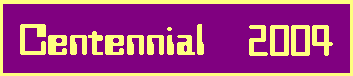# 2-Stage Metabelian 3-Groups

## 1. Commutators Generating the Descending Central Series.

PROPOSITION 1.1.

Assumptions:
Let m,n be rational integers, such that 3 <= m <= n
and let G be a 2-stage metabelian 3-group in ZEF(m,n) with generators x,y.

Claims:
1. G2 = < [y,x],G3 > , i. e., G2/G3 = (3) is cyclic
2. G2 = < [y,x]g | g in G > = [y,x]G , i. e., G2 is a cyclic G/G'-module
3. For all i >= 2, we have the following implication:
if Gi = < g1,...,gr,Gi+1 > with r >= 0 and g1,...,gr in Gi - Gi+1,
then r <= 2i-1 and Gi+1 = < [gj,x],[gj,y],Gi+2 | 1 <= j <= r >
4. In particular, if 4 <= m <= n and if we use the notation s2 = [y,x],
s3 = [s2,x] = s2x-1, t3 = [s2,y] = s2y-1,
then G2 = < s2,G3 > implies that G3 = < s3,t3,G4 >
5. For 4 <= m <= n, there exist generators x,y of G, such that G3 = < x3,y3,G4 > .

## 2. Groups of Maximal Class.

THEOREM 2.1.

Assumptions:
Let m be a rational integer, such that m >= 3,
and let G be a 2-stage metabelian 3-group of maximal class in ZEF(m,m)
with generators s,s1,
where we assume s in G - C2 and s1 in C2 - G' in the case of m > 3, i. e., G' < C2 < G.
Finally, with d(i) = (s - 1)i let si = [s1,s]d(i-2) in Gi for i >= 2.

Claims:
1. The generators satisfy the following relations:
a) relations without s,s1:
si = 1 for i >= m, [si,sj] = 1 for i,j >= 2,
si3si+13si+2 = 1 for i >= 2
b) relations including s1 but without s:
[si,s1] = 1 for i >= 3,
[s2,s1] = sm-1c for some -1 <= c <= 1,
s13s23s3 = sm-1b for some -1 <= b <= 1
c) relations including s:
[si,s] = si+1 for i >= 1,
s3 = sm-1a for some -1 <= a <= 1.
These relations are abbreviated by writing G = G(m)(a,b,c).

2. The structure of the members of the descending central series is given by
Gi = < si > * < si+1 > = A(3,m-i) for all 2 <= i <= m-1,
in particular Gm-1 = < sm-1 > = (3).

 Gm = 1 | Gm-1 = < sm-1 > = (3) | Gm-2 = < sm-2 > * < sm-1 > = (3,3) | ... | G4 = < s4 > * < s5 > | G3 = < s3 > * < s4 > | G2 = < s2 > * < s3 > / | \ M1 C2 = < s1,G2 > M4 \ | / G1 = G = < s,s1 >

## 3. Groups of Non-Maximal Class.

THEOREM 3.1.

Assumptions:
Let m,n be rational integers, such that 4 <= m < n <= 2m - 3,
and put e = n - m + 2 >= 3.
Let G be a 2-stage metabelian 3-group of non-maximal class in ZEF(m,n)
with generators x,y, such that G3 = < x3,y3,G4 >,
where we assume x in G - Cs and y in Cs - G' in the case of s < m - 1, i. e., G in ZEF 2(m,n).
Additionally, with v2 = [y,x], d(x,i) = (x - 1)i, and d(y,i) = (y - 1)i let
vi = [y,x]d(x,i-2), wi = [y,x]d(y,i-2), for i >= 3,
si = (y3)d(x,i-3), ti = (x3)d(y,i-3), for i >= 3,
Si = < sj | j >= i >, Ti = < tj | j >= i >, for i >= 3,
and denote by D the intersection of S4 with T4.

Claims:
1. Gi = < si, ti, Gi+1 > = Si*Ti for i >= 3,
and thus Gi/Gi+1 is at most of 3-rank 2.
2. Si = < si > * < si+1 > ~ A(3,m-i) and #Si = 3m-i for all 3 <= i <= m.
3. If G belongs to ZEF a(m,n), then we have
a) Ti = < ti > * < ti+1 > ~ A(3,e+1-i) and #Ti = 3e+1-i for all 3 <= i <= e+1,
b) the intersection of Si with Ti is trivial (=1),
c) and Gi is the direct product of Si and Ti:
Gi = < si > * < si+1 > * < ti > * < ti+1 >
~A(3,m-i)*A(3,e+1-i) for 3 <= i <= e and ~A(3,m-i) for e+1 <= i <= m.
4. However, if G belongs to ZEF b(m,n), then
a) Ti = < ti > * < ti+1 > ~ A(3,e+2-i) and #Ti = 3e+2-i for all 3 <= i <= e+2,
b) and the intersection of Si with Ti equals
Gm-1 = < sm-1 > = < te+1 > for all 3 <= i <= e+1.

## 4. Transfers ("Verlagerungen").

This will be the topic of our next article.
 References:  Arnold Scholz und Olga Taussky, Die Hauptideale der kubischen Klassenkörper imaginär quadratischer Zahlkörper, J. reine angew. Math. 171 (1934), 19 - 41  Norman Blackburn, On a special class of p-groups, Acta Math. 100 (1958), 45 - 92  Brigitte Nebelung, Klassifikation metabelscher 3-Gruppen mit Faktorkommutatorgruppe vom Typ (3,3) und Anwendung auf das Kapitulationsproblem, Inauguraldissertation, Köln, 1989  Daniel C. Mayer, Principalization in complex S3-fields, Congressus Numerantium 80 (1991), 73 - 87

 <| Navigation Center <| <| Back to Algebra <|